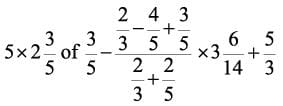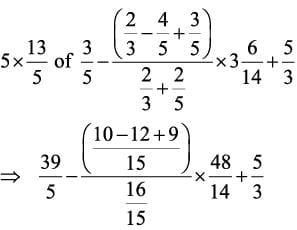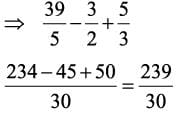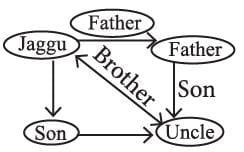Olympiad Test Level 1: Logical Reasoning- 1

# Olympiad Test Level 1: Logical Reasoning- 1

Test Description

## 10 Questions MCQ Test GK Olympiad for Class 6 | Olympiad Test Level 1: Logical Reasoning- 1

Olympiad Test Level 1: Logical Reasoning- 1 for Class 6 2022 is part of GK Olympiad for Class 6 preparation. The Olympiad Test Level 1: Logical Reasoning- 1 questions and answers have been prepared according to the Class 6 exam syllabus.The Olympiad Test Level 1: Logical Reasoning- 1 MCQs are made for Class 6 2022 Exam. Find important definitions, questions, notes, meanings, examples, exercises, MCQs and online tests for Olympiad Test Level 1: Logical Reasoning- 1 below.
Solutions of Olympiad Test Level 1: Logical Reasoning- 1 questions in English are available as part of our GK Olympiad for Class 6 for Class 6 & Olympiad Test Level 1: Logical Reasoning- 1 solutions in Hindi for GK Olympiad for Class 6 course. Download more important topics, notes, lectures and mock test series for Class 6 Exam by signing up for free. Attempt Olympiad Test Level 1: Logical Reasoning- 1 | 10 questions in 20 minutes | Mock test for Class 6 preparation | Free important questions MCQ to study GK Olympiad for Class 6 for Class 6 Exam | Download free PDF with solutions
 1 Crore+ students have signed up on EduRev. Have you?
Olympiad Test Level 1: Logical Reasoning- 1 - Question 1

### What least value should be replaced by * in 5. 223 * 431 so the number becomes divisible by 9?

Detailed Solution for Olympiad Test Level 1: Logical Reasoning- 1 - Question 1

Number is divisible by 9, if sum of all digits is divisible by 9, so (2 + 2 + 3 + * + 4 + 3 + 1) = 15 + * should be divisible by 9, 15 + 3 will be divisible by 9, so that least number is 3.

Olympiad Test Level 1: Logical Reasoning- 1 - Question 2

### What is the largest 4 digit number exactly divisible by 88?

Detailed Solution for Olympiad Test Level 1: Logical Reasoning- 1 - Question 2

Largest 4 digit number is 9999 After doing 9999/88 we get remainder 55 Hence the largest 4 digit number exactly divisible by 88 = 9999 - 55 = 9944

Olympiad Test Level 1: Logical Reasoning- 1 - Question 3

### What least number must be subtracted from 427398 so that remaining number is divisible by 15?

Detailed Solution for Olympiad Test Level 1: Logical Reasoning- 1 - Question 3

On dividing 427398 by 15 we get the remainder 3, so 3 should be subtracted

Olympiad Test Level 1: Logical Reasoning- 1 - Question 4

The value ofDetailed Solution for Olympiad Test Level 1: Logical Reasoning- 1 - Question 4Olympiad Test Level 1: Logical Reasoning- 1 - Question 5

On a scale of map, 2.5 cm represents 30 km. If the distance between two stations on the map is 78.5 cm, then the actual distance between these stations is

Detailed Solution for Olympiad Test Level 1: Logical Reasoning- 1 - Question 5

Required distance = 30/2.5 x 78.5 = 942 km

Olympiad Test Level 1: Logical Reasoning- 1 - Question 6

The students of a class donated ₹2401. Each student donated as many rupees as the number of students in the class, then the number of students in the class is

Detailed Solution for Olympiad Test Level 1: Logical Reasoning- 1 - Question 6

Let the number of students be x.
Amount donated = Rs 2401
According to the given condition
x2 = 2401
x = √2401 = 49 Students.

Olympiad Test Level 1: Logical Reasoning- 1 - Question 7

Pointing to a man, Jaggu said, “His son is my son’s uncle.” How is the man related to Jaggu?

Detailed Solution for Olympiad Test Level 1: Logical Reasoning- 1 - Question 7Olympiad Test Level 1: Logical Reasoning- 1 - Question 8

From the given alternative, select the word which can’t be formed using the letters of the given words.

POSSESSION

Detailed Solution for Olympiad Test Level 1: Logical Reasoning- 1 - Question 8

SESSION → POSSESSION → can be formed.
POSE → POSSESSION → can be formed.
POISE → POSSESSION → can be formed.
OPTICS → POSSESSION → cannot be formed as there is no T, C.

Olympiad Test Level 1: Logical Reasoning- 1 - Question 9

Arrange the fractions in ascending order

Detailed Solution for Olympiad Test Level 1: Logical Reasoning- 1 - Question 9

5/8 = .625
7/12 = .5833
3/4 = .75
13/16 = .8125
So the order will be
7/12 < 5/8 < 3/4 < 13/16

Olympiad Test Level 1: Logical Reasoning- 1 - Question 10

Directions: Select the related word/letter/ number from the given alternatives.

Mathematics : Numbers :: History : ?

Detailed Solution for Olympiad Test Level 1: Logical Reasoning- 1 - Question 10

Mathematics is the science of numbers, quantity and space Similarly, History is the study of past events.

## GK Olympiad for Class 6

22 docs|58 tests
 Use Code STAYHOME200 and get INR 200 additional OFF Use Coupon Code
Information about Olympiad Test Level 1: Logical Reasoning- 1 Page
In this test you can find the Exam questions for Olympiad Test Level 1: Logical Reasoning- 1 solved & explained in the simplest way possible. Besides giving Questions and answers for Olympiad Test Level 1: Logical Reasoning- 1, EduRev gives you an ample number of Online tests for practice

22 docs|58 tests